Courses

# Test: Acceleration due to Gravity

## 10 Questions MCQ Test Physics Class 11 | Test: Acceleration due to Gravity

Description
This mock test of Test: Acceleration due to Gravity for Class 11 helps you for every Class 11 entrance exam. This contains 10 Multiple Choice Questions for Class 11 Test: Acceleration due to Gravity (mcq) to study with solutions a complete question bank. The solved questions answers in this Test: Acceleration due to Gravity quiz give you a good mix of easy questions and tough questions. Class 11 students definitely take this Test: Acceleration due to Gravity exercise for a better result in the exam. You can find other Test: Acceleration due to Gravity extra questions, long questions & short questions for Class 11 on EduRev as well by searching above.
QUESTION: 1

Solution:
QUESTION: 2

### The mass of a planet is 5 times the mass of the earth but its diameter is the same as that of the earth. How much work is done in lifting a stone of mass 3 kg through a distance of 1 m on this planet?

Solution:

We know that g∝Mp​, Where Mp​ is mass of planet.

So,  gp​=5×10=50 m/s2

So work done in lifting a 3 kg stone by 1m =50×3×1=150J

QUESTION: 3

### The earth is an approximate sphere. If the interior contained matter which is not of the same density everywhere, then on the surface of the earth, the acceleration due to gravity :

Solution:

There's no such thing as zero gravity. Weightlessness and zero gravity are two different things. The earth's gravity keeps the moon in orbit. And astronauts are generally much closer to earth than the moon is, which means that the earth's pull on them has to be much stronger.

QUESTION: 4

The value of acceleration due to gravity on earth is.

Solution:

The value of acceleration due to gravity on earth is g=9.8ms−2.

Hence,

QUESTION: 5

The earth's gravitational force at someplace in space causes an acceleration of 7m/s2 in a 1kg mass.What will be the acceleration of a 5kg mass at the same place?

Solution:

The acceleration due to gravity does not vary with the change in the mass of the object as it is a dimensionless quantity representing the amount of matter in a particle or object.
Therefore, the acceleration of a 5 kg mass at the same place is 7m/s2.

QUESTION: 6

A ball is thrown vertically upwards. The acceleration due to gravity:

Solution:

A ball is thrown vertically upwards. The acceleration due to gravity is in the downward direction.

QUESTION: 7

Radius of planet A is twice that of planet B and the dencity of A is one third that of B . The ratio of the acceleration due to gravity at the surface of A to that at the surface of B is.-

Solution:

Radius of planet A is twice that of planet B and the density of A is one-third that of B.The density is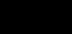We know that,

The acceleration due to gravity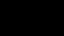The ratio of the acceleration due to gravity at the surface of A to that at the surface of B is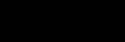We know,

Mass = density X Volume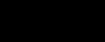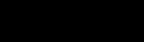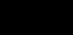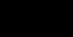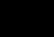Hence, the ratio of the acceleration due to gravity is 2:3.

QUESTION: 8

The diameters of two planets are in the ratio 4:1 and their mean densities in the ratio 1:2. The acceleration due to gravity on the planets will be in ratio

Solution:
QUESTION: 9

Force of gravity is least at

Solution:
QUESTION: 10

Two balls are dropped from the same height from places A and B. The body at B takes two seconds less to reach the ground at B strikes the ground with a velocity greater than at A by 10m/s. The product of the acceleration due to gravity at  the two places A and B is:

Solution: